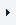LOGINREGISTER (FREE TRIAL)myTOMLAB
 ProductsTOMLAB Base ModuleTOMLAB /MINOSTOMLAB /NPSOLTOMLAB /DNOPTTOMLAB /SNOPTTOMLAB /SOLTOMLAB /CGOTOMLAB /CPLEXTOMLAB /GUROBITOMLAB /MINLPTOMLAB /MIPNLPTOMLAB /MISQPTOMLAB /PENSDPTOMLAB /PENBMITOMLAB /KNITROTOMLAB /OQNLPTOMLAB /CONOPTTOMLAB /PROPTTOMLAB /NLPQLTOMLAB /LGOTOMLAB /LGO-MINLPTOMLAB /GPTOMLAB /GENOTOMLAB /MADTOMLAB /MIDACO

# TOMLAB /MAD

TOMLAB /MAD is a full TOMLAB integration of MAD (MATLAB Automatic Differentiation) from Cranfield University.

The user of TOMLAB /MAD may specify if the 1st or 2nd derivatives of the objective function and constraints should be evaluated. The gradient and constraint derivatives must be given when obtaining the 2nd derivatives, i.e. one level of differentiation is supported. To use MAD after successful installation, simply set Prob.ADObj = 1 and Prob.ADCons = 1. If the 2nd derivatives are to be calculated by MAD set Prob.ADObj = 2 and Prob.ADCons = 2.

MAD is a MATLAB library of functions and utilities for the automatic differentiation of MATLAB functions and statements. MAD utilizes an optimized class library "derivvec", for the linear combination of derivative vectors.

## Main features

• A new MATLAB class "fmad" which overloads the builtin MATLAB arithmetic and some intrinsic functions.
• Separation of storage and manipulation of derivatives into a separate class "derivvec". This greatly reduces the complexity of the MATLAB code for the "fmad" class. Additionally it allows for the straight forward optimization of the derivative vector operations using the high level matrix and array functions of MATLAB.
• The "derivvec" class also allows for the use of MATLAB's sparse matrix representation to exploit sparsity in the derivative calculation at runtime.

Coverage of MATLAB's huge range of intrinsic functions is currently limited. New functionality will be added as demanded by users allowing it to be carefully tested on user's test cases before widespread release.

Also see http://www.amorg.co.uk/AD/MAD/ and http://www.amorg.co.uk/AD/PUBS/saf_toms06.htm for more information.

### Requirements:

MATLAB 6.5 or later.

Tomlab © 1989-2018. All rights reserved.    Last updated: May 24, 2018. Site map. Privacy Policy